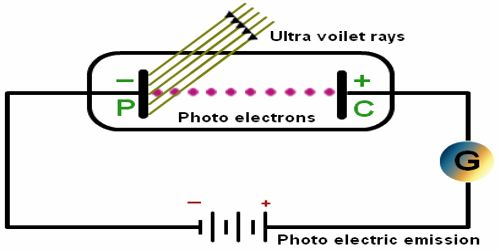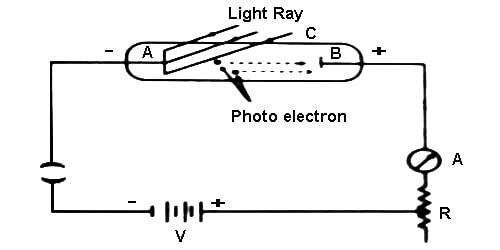# Experiment: Demonstration of Photoelectric Effect

Experiment: Demonstration of Photoelectric Effect

The photoelectric effect refers to the emission, or ejection, of electrons from the surface of, generally, a metal in response to incident light. Perform this experiment using a quartz tube, two zinc plates, cathode and anode plates, ammeter, key, a ring and a variable resistor. In this experiment C is an evacuated quartz tube. There are two plates of zinc inside the tube. One is A and the other one is B. A coating of alkali metal of sodium or potassium or lithium etc is there in the plate A. In that experiment a coating of lithium dioxide (Li2O) is there on this plate A. The plate A is connected to the negative terminal of the battery and the plate B, an ammeter and a variable resistor R are connected to the positive terminal of the battery (Figure). By changing the value of R, the potential difference between the plates is collected.Now, when the light is incident on the plate A, electrons are emitted. Since plate B is positive, so electrons will be attracted by it and current will flow in the circuit. As a result, the ammeter will show deflection. If no light is incident on the plate, current will not flow. The magnitude of current will depend on the intensity of the incident light. Again keeping the intensity constant, if the potential difference is increased, current will also increase, but for a particular potential difference, current will reach to a fixed value. Afterward, if the potential difference is increased further, current will remain unchanged.

Now, let us keep A at positive and B at negative potentials. If the light is incident on A, emitted electrons will be attracted by A and current will decrease. If the positive potential of A is gradually increased, current will continue to decrease and at a fixed potential current will be zero. This potential is called the stopping potential. The stopping potential does not depend on the intensity of the incident light; but it depends, on the frequency of tine incident light. Besides, it depends on the nature of the material. From the experiment, it has been observed that below a fixed value of the frequency of the incident light, no electrons emit from the metal. This frequency is called threshold frequency [Figure].

From the above discussion stopping potential, threshold frequency and work function may be defined in the following way.

Threshold frequency: For every metal, there is a minimum frequency below that frequency of light electrons from that metal surface cannot be emitted. That minimum frequency is called threshold frequency of that metal.

Stopping potential: The minimum negative potential applied on anode plate with respect to the cathode plate for which the photoelectric current just stops is called stopping potential.

Work function: The amount of energy needed to emit electron having zero velocity from the surface of a metal is called the work function of that metal.